# Creating an Interactive Statistical Distribution Graph in Excel 2010 and Excel 2013

This is one of the following eleven articles on creating user-interactive graphs of statistical distributions in Excel

Interactive Statistical Distribution Graph in Excel 2010 and Excel 2013

Interactive Graph of the Normal Distribution in Excel 2010 and Excel 2013

Interactive Graph of the Chi-Square Distribution in Excel 2010 and Excel 2013

Interactive Graph of the t-Distribution in Excel 2010 and Excel 2013

Interactive Graph of the t-Distribution’s PDF in Excel 2010 and Excel 2013

Interactive Graph of the t-Distribution’s CDF in Excel 2010 and Excel 2013

Interactive Graph of the Binomial Distribution in Excel 2010 and Excel 2013

Interactive Graph of the Exponential Distribution in Excel 2010 and Excel 2013

Interactive Graph of the Beta Distribution in Excel 2010 and Excel 2013

Interactive Graph of the Gamma Distribution in Excel 2010 and Excel 2013

Interactive Graph of the Poisson Distribution in Excel 2010 and Excel 2013

Interactive area charts are very useful for demonstrating how changes made to a statistical distribution’s parameters affect the distribution’s shape, scale, and location.

This section shows how to create a user-interactive normal distribution graph in Excel that instantaneously adjusts its shape, scale, location, and size of outer tails when a user changes any of the yellow cells that contain the graph’s parameters, label and legend text, and percentage of curve area in each outer tail. The following user-controlled settings produce the Excel area chart that is shown here:(Click On Image To See a Larger Version)

Changes to each of the nine user-controlled settings instantly update the chart as shown here:(Click On Image To See a Larger Version)

This section will provide instructions to create this interactive Excel area chart.

The X and Y values used to create this chart are based upon z Score(X), the z Score of each X value. The z Score is the number of standard deviations that a specific point (X value) is from the mean.

The z Score provides the basis of every element on this chart and therefore should be constructed first. 99.7 percent of all values in a normally distributed population will be within 3 standard deviations from the mean. A sufficient range of z Score values would include z Scores from 3 standard deviations below the mean (z Score = -3) to 3 standard deviations above the mean (z Score = +3).

The z Score data will be presented as a list of z Scores starting at -3 and increasing incrementally to a value of +3. The increments need to be small enough so that Excel graph based upon these z Scores will be smooth. The list of z Scores will increase by increments of 0.10 from -3 to +3. This means that there be 60 z Score values and therefore 60 points graphed in this Excel chart.

As mentioned the z Score will start at -3 and increase in 0.1 increments until the z Score has reached +3. There are many ways in Excel to incrementally increase the values in cells on the way down a column. ROW() generates the number of the current row and is used in the following fashion to incrementally increase z Score value:(Click On Image To See a Larger Version)

The Excel Split View tool was used to simultaneously view the beginning and the end of a data column. The beginning and end values of data columns are usually the most important parts when creating interactive Excel graphs. The Split View tool is found under the View tab and is labeled Split. The horizontal and vertical partitions of the Split can be dragged-and-dropped to any location on the worksheet by the user.

The next set of graph parameters that should be created are the X-axis values. The values of the X-axis are calculated from the z Score, the population mean (µ) and the population standard deviation (σ). The calculation of the X values on the horizontal axis is given as follows:

X = µ + (z Score(X) * σ)

z Score data has already been created. The population mean, µ, and population standard deviation, σ, will be tied to user-inputs on the Excel worksheet.

Changing the Excel graph’s user inputs µ and σ changes the values of the X-axis. The graph’s shape remains the same but the values of the X axis are shifted and scaled based upon changes made to the user inputs µ and σ. The two graphs at the beginning of this section show the changes in the X-axis location and scale based upon changes to user inputs for µ and σ. The following image shows how X-axis values on the Excel worksheet are calculated based upon z Scores and the user inputs µ and σ:(Click On Image To See a Larger Version)

After creating the column of z Scores and the column of X-axis values tied to the z Scores, the next step is to create a column of PDF data. The z Score data is the fundamental basis of the entire graph and each PDF value in the PDF column is based upon the z Score that is directly across from it in the z Score column.

These PDF values are calculated in Excel as follows:

PDF(X)

= f(X,µ,σ)

=NORM.DIST(X, µ,σ,FALSE)

= NORM.S.DIST(z Score(X),FALSE)

= NORM.S.DIST((X - µ)/σ,FALSE)

This is implemented in the Excel worksheet as follows:(Click On Image To See a Larger Version)

The next data set that should be created are the values of the normal distribution’s CDF (Cumulative Distribution Function) at each z Score increment. This is calculated in Excel as follows:

CDF(X)

= F(X,µ,σ)

=NORM.DIST(X, µ,σ,TRUE)

= NORM.S.DIST(z Score(X),TRUE)

= NORM.S.DIST((X - µ)/σ,TRUE)(Click On Image To See a Larger Version)

The data sets created so far include z Score values, X-axis values, and the PDF and CDF values at each z Score. The final data set to be created are the Y-axis values.

This Excel area chart is a graph of the normal distribution’s bell-shaped PDF curve. The Y value at each point on this curve will therefore have the same value as the normal distribution’s PDF at that point. Setting the Y-values to equal the PDF values for each z Score increment is implemented as follows:(Click On Image To See a Larger Version)

The Y-values are given the label Series 1 because this represents the first data series created for the area chart.

Series 1 can now be graphed because both the X and Y values are now available. Creating this graph in Excel is done as follows:

The first step is to select the entire column of data including the label for Series 1 as shown.

With that selected data highlighted, insert an area chart into the worksheet. That chart is inserted by going under the Insert tab and then selecting Area Charts / 2-D Area Chart as follows:(Click On Image To See a Larger Version)

The following graph of the normal distribution’s PDF is automatically created as follows:(Click On Image To See a Larger Version)

The default color of the first data series graphed is blue. This can be changed if desired by right-clicking on blue bell-shaped curve and selecting Format Graph Area.

The area chart has been created based upon the column of Y values. No specific values have been assigned to the X-Axis. The default X values are created from the numbering of the points starting at 1 on the left.

The next step is to assign specific X-values to the data points. The X-value data set column has been created.

To assign specific X-values to the graph’s data points, click anywhere on the graph and choose Select Data from the pop-up short-cut menu as follows:(Click On Image To See a Larger Version)

This brings up the Select Data Source dialogue box. Select the Edit button on the right side under Horizontal (Category) Axis Labels.(Click On Image To See a Larger Version)

This will bring up the Axis Label dialogue box. Highlight the data in the X-Axis data column on the worksheet and hit OK as follows:(Click On Image To See a Larger Version)

The Excel graph will now attach the selected X values to the data points as follows:(Click On Image To See a Larger Version)

These X-values change instantly when the user inputs for µ and σ are changed. This will be demonstrated shortly.

The values in the X-axis should have additional formatting applied to them. The X axis can be made more legible if the X-axis values have their font weight changed to Bold, are set to show at least one decimal place, and become spaced out in larger increments than the current crowded setting of displaying than every third X value. These changes can be made to the X–axis values as follows:

Right-click anywhere on the X-axis to bring up both the short-cut font menu and the short-cut pop-up menu. Select B on the short-cut font menu and the X values will have their font weight changed to bold.

Right-click again on the numbers on the X-axis to bring up both menus again. Select Format Axis as follows:(Click On Image To See a Larger Version)

This will bring up the Format Axis dialogue box. Select the first option on the left which is Axis Options. The following settings shown here will display every tenth X-axis value instead of every third value:(Click On Image To See a Larger Version)

The Number category brings up the Number dialogue page. The following setting will configure the X values to display one decimal place:(Click On Image To See a Larger Version)

These settings configure values on the X axis to be displayed as follows:(Click On Image To See a Larger Version)

As mentioned, this graph is user-interactive. Changing user-inputs µ and σ produce the following changes in the Excel graph:(Click On Image To See a Larger Version)

The graph’s shape did not change. The location of the mean was shifted to 50 and the graphed was scaled so that its standard deviation is now equal to 20. These changes are all implemented in the X axis.

Excel graphs can be configured to link the text of the chart title and axis labels to external cells that are user inputs. The first step to implementing this is to create the external cells that will be the user inputs. Place these inputs in the following locations: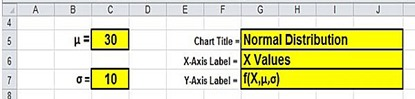(Click On Image To See a Larger Version)

The current generic Excel area chart does not have axis labels. The labels for the X-axis and the Y-axis have to created.

A generic X-axis label is created by first clicking anywhere on the chart. This will bring up the Chart Tools menu. From this menu select the Layout tab / Axis Titles / Primary Horizontal Axis Title / Title Below Axis.(Click On Image To See a Larger Version)

This will create a generic X-axis title on the bottom of the graph shown in the next image of the graph.

A generic Y-axis label is also created by first clicking anywhere on the chart. This once again bring up the Chart Tools menu. From that menu select the Layout tab / Axis Titles / Primary Vertical Axis Title / Horizontal Title.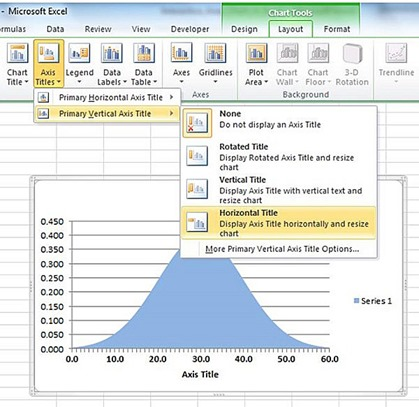(Click On Image To See a Larger Version)

This will create a generic Y-axis title on the left side of the graph that has horizontal text that is shown in the next image of the graph.

The Excel chart is now showing generic X-axis and Y-axis labels, a chart title, and a legend for data Series 1.(Click On Image To See a Larger Version)

Linking the text in the chart title to text in an external user-input cell is fairly simple. Just click anywhere on the chart title and then type an equal sign in the formula bar as follows:(Click On Image To See a Larger Version)

After typing the equal sign in the formula bar, click on the external cell that contains text. The address of this cell will appear in the formula bar including the worksheet’s name as follows: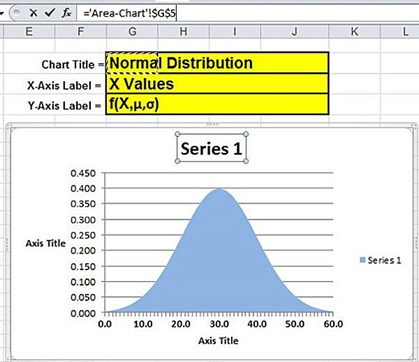(Click On Image To See a Larger Version)

The result is that the chart title now displays the text contained in the external cell as follows:(Click On Image To See a Larger Version)

If the text in the external cell is changed, the updated text will be immediately displayed in the Excel chart.

That exact same procedure can be used to link the X and Y-axis labels to external cells that are controlled by the user. Performing this procedure for both labels produces the following result:(Click On Image To See a Larger Version)

The next step is to link the legend of data Series 1 to an external cell. To do this click anywhere on the chart to bring up the short-cut menu. From this menu, choose Select Data as follows:(Click On Image To See a Larger Version)

This will bring up the Select Data Source dialogue box. Select Series 1 on the left side and then click the Edit button also on the left side as follows:(Click On Image To See a Larger Version)

This will bring up the Edit Series dialogue box. Under the Series name input shown below in this dialogue box, select the external cell containing the text for the legend as follows:(Click On Image To See a Larger Version)

The preceding steps have produced an Excel area chart that has the chart title, the labels for the X and Y axes, and the legend for Series 1 linked to external cells that can each be changed by the user as follows: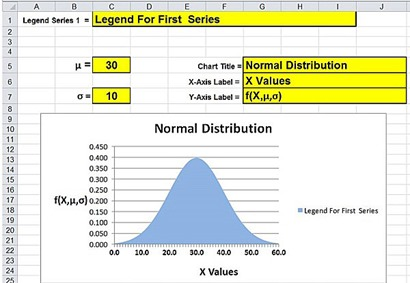(Click On Image To See a Larger Version)

Any changes in these user inputs are instantly reflected in the Excel graph as follows:(Click On Image To See a Larger Version)

The next step is to create an additional data series that will display the outer tails of the graph. This data series will have its data values controlled by the user inputs. These inputs will control the curve area displayed in each of the outer tails of the graph.

The first step in creating this data series (Series 2) is to add new user inputs as follows:(Click On Image To See a Larger Version)

The data values for Series 2 can now be created.

The Y values of Series 2 will also be set to equal the PDF values at each point on the graph as follows:(Click On Image To See a Larger Version)

Now that the column of Y values for Series 2 has been created, Series 2 can be added to the area chart and linked to the column of Y values. To do that click anywhere on the chart to bring up the pop-up short-cut menu. Choose Select Data on the menu as follows: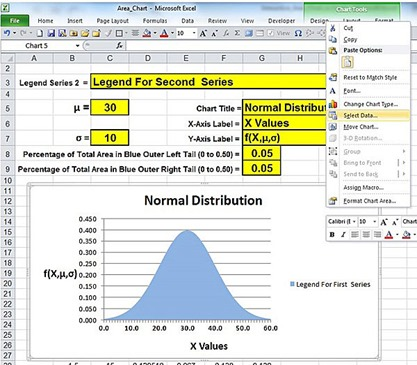(Click On Image To See a Larger Version)

This will bring up the following Select Data Source dialogue box. Select Add on the left side under Legend Entries (Series).(Click On Image To See a Larger Version)

For the Series name data input box, click on the external cell that contains the text that will be the legend for Series 2.(Click On Image To See a Larger Version)

From the Series Values input box, select the column of Series 2 Y values on the worksheet as follows:(Click On Image To See a Larger Version)

Any data series that is added to an Excel area chart will sit on top of all previously-added ones. Series 2 (default color is red) sits on top of Series 1 (default color is blue) and completely covers Series 1 on the chart as follows:(Click On Image To See a Larger Version)

Series 2 sits on top of and covers Series 1 on the chart. The only way to see any of the blue Series 1 is to zero out Y values of Series 2.

For example, if the following outer values of Series 2 were set to zero:(Click On Image To See a Larger Version)

The blue Y values of Series 1 underneath are uncovered and show through as the outer tails on each side. The resulting chart from the above data is shown as follow:(Click On Image To See a Larger Version)

It is possible to create a user input that automatically determines how many outer Y values on each side of Series 2 will be set to zero. The number of Y values set to zero on each side of Series 2 determines how much curve area each blue outer tail in the graph will contain.

Each outer tail is addressed separately and has a separate user input assigned to it. Each of the two user inputs specify the percentage of the total curve area that will be displayed as a blue outer tail on its respective side.

The upper user input set to the value of 0.05 controls the outer left tail. The user-controlled value of 0.05 specifies that the blue outer left tail will contain 5 percent of the total area under the curve.

Each of the Y values in Series 2 is calculated in Excel by an If-Then-Else statement. The If-Then-Else statement sets the Y value to zero if the user input (currently set at 0.05 for both tails) is equal or greater than its respective CDF value (in the left tail) or 1 – CDF (in the right tail).

When the above condition is not met, the If-Then-Else statement sets the Y value to its respective PDF value. The Y value will either be set to zero (if the condition is met) or set to its PDF value (if the condition is not met).

The If-Then-Else formula is changed when the z Score reaches zero. This formula change ensures that the user input for each of the two outer tails will always be compared to the area in that outer tail.

The CDF(X) states the percentage of the total bell-shaped normal curve is to the left of X. If point X is in the left tail, the curve area outside of point X in the left tail is specified by CDF(X). If point X is in the right tail, the curve area outside of point X in the right tail is specified by 1 – CDF(X).

The following image shows the formula change at the point that the z Score equals zero. The Excel formulas for the Y values of Series 2 change when the z Score reaches zero. Y values in the left tail have the tan background. Y values in the right tail have the light blue background.(Click On Image To See a Larger Version)

As a result, the settings shown below automatically configure the graph to display blue outer left and right tails that each contain 5 percent of the total area under the normal curve.

This was implemented by setting the Y values of Series 2 to zero in the outer 5 percent of each tail. This enables the blue Y values of Series 1 underneath to be displayed in those outer tails.(Click On Image To See a Larger Version)

Changes to any of the yellow user inputs are automatically reflected in the Excel graph.

The following graph shows how the changes made to all nine user inputs are instantly and automatically reflected in the Excel area graph as follows:(Click On Image To See a Larger Version)

Excel Master Series Blog Directory

Statistical Topics And Articles In

This Blog

You Will Become an Excel Statistical Master!

1.thanks for this, by the way I bought all your e-books a few years ago, do they come with updates from excel 2007, to later versions

2.ارخص شركة نقل عفش

ارخص شركة نقل عفش بالمدينة المنورة نقل عفش بالمدينة المنورة
ارخص شركة نقل عفش بمكة نقل عفش بمكة
شركة نقل عفش شركة نقل عفش

ارخص شركة نقل عفش بجدة نقل عفش بجدة
دينا نقل عفش جدة دينا نقل عفش بجدة